Next: HOUSEHOLDER TRANSFORMATIONS AND GOLUB'S Up: Data modeling by least Previous: WEIGHTS AND CONSTRAINTS

# FEWER EQUATIONS THAN UNKNOWNS

What is one to do when one has fewer equations than unknowns: give up? Certainly not, just apply the principle of simplicity. Let us find the simplest solution which satisfies all the equations. This situation often arises. Suppose, after having made a finite number of measurements one is trying to determine a continuous function, for example, the mass density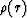as a function of depth in the earth. Then, in a computerwould be represented bysampled at N depths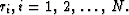Then merely taking N large, one has more unknowns than equations.

One measure of simplicity is that the unknown function xi has minimum wiggliness. In other words minimize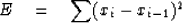(42)

subject to satisfying exactly the observation or constraint equations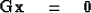(43)

Another more popular measure of simplicity (which does not imply an ordering of the variables xi) is the minimization of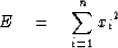(44)

If we set out to minimize (44) without any constraints, x would satisfy the simultaneous equations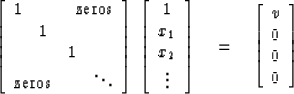(45)

By inspection one sees the obvious result that xi = 0. Now let us include two constraint equations and, for definiteness, take three unknowns. The method of the previous section gives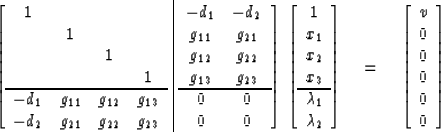(46)

Equation (46) has a size equal to the number of variables plus the number of constraints. It may be solved numerically or it may be reduced to a matrix whose size is given by the number of constraints. Let us split up (46) into two equations: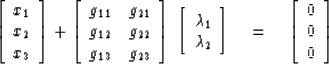(47)
and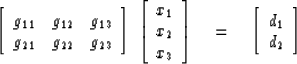(48)

We abbreviate these equations by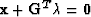and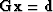,Premultiply (47) by G,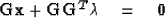insert (48)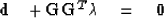solve for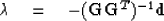put back into (47)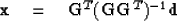Written out in full this is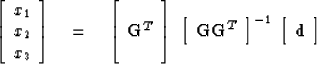(49)

This is the final result, a minimum wiggliness solution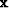which exactly satisfies an underdetermined set called the constraint equations.

## EXERCISES:

1. If wiggliness is defined by (42) instead of (44), what form does (49) take?
2. Given the mass and moment of inertia of the earth, calculate mass density as a function of depth utilizing the principle of minimum wiggliness (49). What criticism do you have of this procedure? (HINT: An elegant solution uses integrals instead of infinite sums.)
3. Use the techniques of this section on (40) to reduce the size of the matrices to be inverted.Next: HOUSEHOLDER TRANSFORMATIONS AND GOLUB'S Up: Data modeling by least Previous: WEIGHTS AND CONSTRAINTS
Stanford Exploration Project
10/30/1997